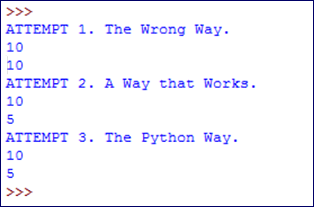# How to do Simultaneous Assignment in Python

See Python: Tips and Tricks for similar articles.

A very cool feature of Python is that it allows for simultaneous assignment. The syntax is as follows:

``var_name1, var_name2 = value1, value2``

This can be useful as a shortcut for assigning several values at once, like this:

``smart_1, cute_1, funny_1, quiet_1 = "John","Paul","Ringo","George"``

But simultaneous assignment is really useful in a scenario like the one shown below.

1. First, let's look at a method that does not work:
``````a=5
b=10
a=b
b=a
print("ATTEMPT 1. The Wrong Way.")
print(a)
print(b)``````
2. Next, let's look at a method that works, but is not Pythonic:
``````a=5
b=10
temp=a
a=b
b=temp
print("ATTEMPT 2. A Non-Pythonic Way that Works.")
print(a)
print(b)``````
3. Finally, let's see the Pythonic way, which is to use simultaneous assignement:
``````a=5
b=10
a,b = b,a
print("ATTEMPT 3. The Python Way.")
print(a)
print(b)``````

The output should look like this:The code demonstrates that simultaneous assignment really is simultaneous. The value for `a` does not change before the value of `b`, so `b` gets the original value of `a`, and we can switch the values without using an interim step of creating a temporary (and otherwise useless) variable.